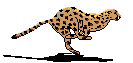Almost every time I'm debugging some core part of our framework, I like to see the generated asm, and trying to optimize the pascal code for better speed - when it is worth it, of course!
I just made a nice observation, when comparing the assembler generated by Delphi to FPC's output.Imagine you compile the following lines (extracted from SynCommons.pas), to convert some number into ASCII characters:

```...
c100 := val div 100;
dec(val,c100*100);
PWord(P)^ := TwoDigitLookupW[val];
...
```

This divides a number by 100, then computes the modulo in `val`, to store two digits at a time. We did not use `val := val mod 100` here, since `mod` would do another division, so we rely on a simple multiplication to compute the modulo.
You may know that for today's CPUs, integer multiplication is very optimized, taking a cycle (or less, thanks to its pipelines), whereas a division is much more expensive - if you have some spare time, take a look at this document, and you will find out that a `div` opcode could use 10 times more cycles then a `mul` - even with the latest CPU architectures.
Let's see how our two beloved compilers do their homework (with optimization enabled, of course)...

Delphi generates the following code for ```c100 := val div 100```:

```005082AB 8BC1             mov eax,ecx
005082B2 33D2             xor edx,edx
005082B4 F7F6             div esi
```

Whereas FPC generates the following:

```0043AC48 8b55f8                   mov    -0x8(%ebp),%edx
0043AC4B b81f85eb51               mov    \$0x51eb851f,%eax
0043AC50 f7e2                     mul    %edx
0043AC52 c1ea05                   shr    \$0x5,%edx
0043AC55 8955f0                   mov    %edx,-0x10(%ebp)
```

Even if you are assembler agnostic, and once you did get rid of the asm textual representation (Delphi uses Intel's, whereas FPC/GDB follows AT&T), you can see that Delphi generates a classic (and slow) `div esi` opcode, whereas FPC uses a single multiplication, followed by a bit shift.

This optimization is known as "Reciprocal Multiplication", and I would let you read this article for mathematical reference - or this one.
It multiplies (`mul`) the number by the power of two reciprocal of 100 (which is the hexadecimal `51eb851f` value), followed by a right shift (`shr`) of 5 bits.
Thanks to 32 bit rounding of the integer operations, this would in fact divide the number per 100.
Even it consists in two assembler opcodes, a `mul` + `shr` is in fact faster than a single `div`.

It is a shame that the Delphi compiler did not include this very common optimization, which is clearly a win for some very common tasks.

Of course, the LLVM back-end used on the NextGen compiler can do it, be we may expect this classic optimization be part of the decades-old Delphi compiler.
And I'm still not convinced about the performance of the NextGen generated code, since the associated RTL is known to be slow, so won't benefit of LVVM optimization - which takes  a LOT of time to compile, by the way (much more than FPC).

Congrats, FPC folks!